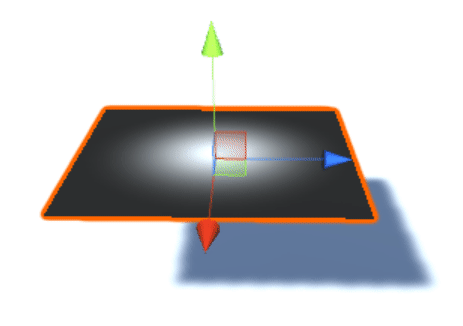I wrote wind shader that works but as you can see it have shadow problem! when I animating vertices shadow is fixed!Shader "Smkgames/Wind Shader" {
Properties{
_MainTex("Albedo and alpha (RGBA)", 2D) = "white" {}
_BumpTex("BumpTex",2D) = "bump"{}
_WindMap ("WindMap", 2D) = "white" {} // Add noies map like fbm noises
_Cutoff ("Cutoff", Range(0,1)) = 0.5
_Speed("Speed",float) = 1
_Direction("Direction",Vector) = (0,0.2,0,0)
}

Cull Off

Tags{ "Queue" = "Transparent" "IgnoreProjector" = "True" "RenderType" = "TransparentCutout" "DisableBatching"="True" }
LOD 200

CGPROGRAM
#pragma surface surf Standard alphatest:_Cutoff vertex:vert

#pragma target 3.0

sampler2D _MainTex,_WindMap,_BumpTex;
fixed4 _Direction;
fixed _Speed, _WindAmount;
half _Glossiness, _Metallic;
fixed4 _Color;

void vert(inout appdata_full v) {
float4 tex = tex2Dlod(_WindMap, float4(v.texcoord.xy + (_Time.x * _Speed), 0, 0));
v.vertex.xyz += tex.y *  _Direction.xyz * _Speed;
}

struct Input {
float2 uv_MainTex;
float2 uv_BumpTex;
};

void surf(Input IN, inout SurfaceOutputStandard o) {
fixed4 c = tex2D(_MainTex, IN.uv_MainTex);
o.Albedo = c.rgb;
o.Alpha = c.a;
o.Normal = UnpackNormal(tex2D(_BumpTex,IN.uv_BumpTex));
}
ENDCG
}
FallBack "VertexLit"
}


I couldn't fix it! so I tried to add shadow to my object from scratch by using vertex and fragment shader shadow caster and worked correctly but I want know Is there a way to fixing this problem in surface shader?

Just add "addshadow" to your #pragma surface surf Standard alphatest:_Cutoff vertex:vert line. Boom Unity does the rest. So it looks like this :
#pragma surface surf Standard alphatest:_Cutoff vertex:vert addshadow